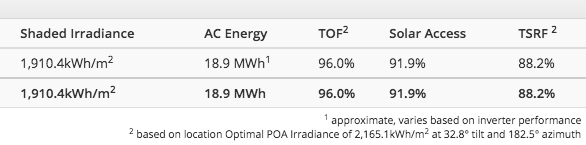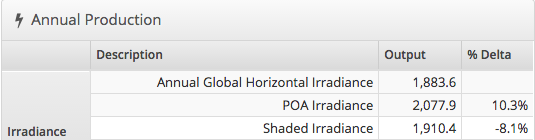# Total Solar Resource Factor (TSRF)

HelioScope Shade Reports produce a variety of outputs. One of the key metrics that the solar industry has come to use for validating proper design is the Total Solar Resource Factor or TSRF. Fundamentally, TSRF is a comparison between the productivity of an actual design to the ideal design for a given location.

There are two components to the calculation of TSRF.

1. The orientation of the modules (Tilt and Orientation Factor)
2. The shade obstructing the modules (Solar Access %)

To start, HelioScope calculates the available irradiance at the ideal tilt and orientation for the project location. This value is expressed in kWh/m2 in the shade report as "Optimal POA". Note: POA stands for Plane of Array, which is the available irradiance after factoring in tilt and azimuth of the design.

In the example below the Optimal POA is 2165.1 kWh/m2 with an ideal tilt of 32.8 and an ideal azimuth of 182.5.To find the Tilt and Orientation Factor (TOF) HelioScope compares the POA Irradiance of each module to the Optimal POA Irradiance value. If we evaluate the POA Irradiance in the Production Report for the design above we see the actual design is getting a POA Irradiance of 2077.9 kWh/m2 vs the Optimal POA of 2165.1 kWh/m2 or 96%, which matches the TOF value in the report.To find Solar Access % HelioScope compares the Shaded Irradiance (1910.4 kWh/m2) value to the POA Irradiance (2077.9 kWh/m2) which gives 91.9%.

To find Total Solar Resource Factor (TSRF) HelioScope compares Shaded Irradiance (1910.4 kWh/m2) to the Optimal POA Irradiance (2165.1 kWh/m2) which gives 88.2%. Another way to calculate TSRF is to multiply the TOF by the Solar Access % (96% x 91.9% = 88.2%). Both methods of calculating TSRF give the same value.